# Gas Liquid Separation

Gas liquid separation is often based on the principle of gravity settling, when liquid droplets suspended in rising gas vapors settle down at the bottom of the separation vessel and are eventually taken out through the bottom. Gas stream separated from liquid is taken out from the top of the separation vessel. The separation of liquid droplets from vapor phase can be explained with the help of following equation for terminal velocity of liquid droplets.where,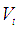= Terminal / critical velocity of gas so that a liquid droplet of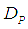size can settle out of the gaseous stream= Liquid droplet diameter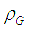= Vapor phase densities respectively= Gravitational acceleration

The drag coefficient is a function of the Reynolds number for liquid droplet which is expressed as,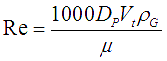The vapor phase density and viscosity are to be used for determining the Reynolds number.

Based on these equations, simpler equations and correlations have evolved for sizing of horizontal and vertical gas-liquid separators.

Most vertical separators are sized based on the following two equations which have been developed using the terminal velocity equation and the drag coefficient expressed as a function of Reynolds number.

For vertical separators,..............................................(A)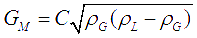...................................(B)

where,

K and C are sizing factors for gas-liquid separators with C=3600K.is the gaseous mass flow divided by the cross sectional area.

Equations (A) and (B) are essentially the same and (B) can be obtained by multiplying both sides of (A) by vapor density.

For smaller horizontal vessels (length less than 3m), equations (A) and (B) can be used for sizing. But for vessels larger than that, equations (C) and (D) have to be followed.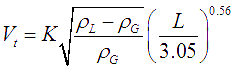............................(C)

For horizontal and vertical vessels, the terminal velocity can be found by using the equations presented above and the sizing factors commonly used in industry. The minimum length can be determined by equating the time required for the liquid droplet (with minimum diameter to be separated) to settle down with the time for the gas stream to pass from inlet to the vapor outlet. To perform this length calculation, vessel diameter must be fixed before. Usually gas-liquid separators have an L/D ratio ranging from 2:1 to 4:1. A choice of length and diameter satisfying the sizing equations can be made to fall in this acceptable range for L/D ratio.